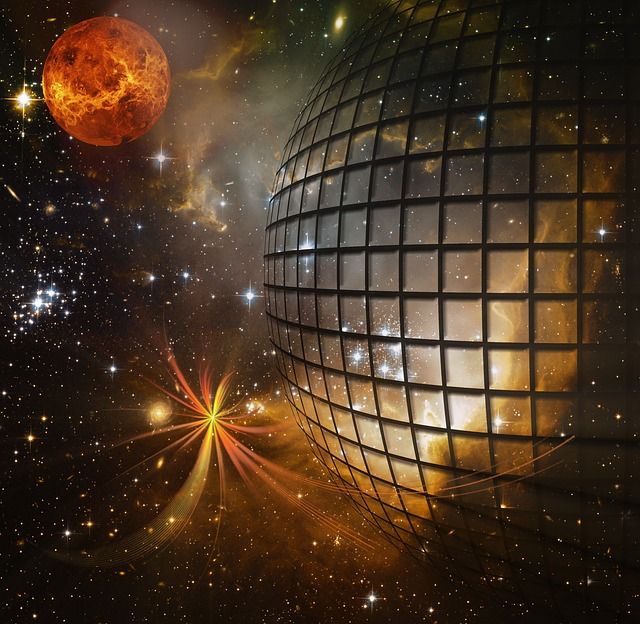Digital Tbucket Tank (DTT)

# How heavy can a graviton be?Scientists are trying to determine the properties of  gravitons to determine - of a hypothetical particle, the one gravitational interaction exercises in an im Journal of High Energy Astrophysics In their published work, Prof. Marek Biesiada and colleagues found a new constraint on the mass of the galaxy from an analysis of 12 galaxy clusters gravitons derived. It is seven orders of magnitude stronger than the limitations resulting from the observations of the  Gravitational waves result.

General Relativity (GRT) changed our ideas about gravity. After the ART curves matter space-time, and all objects move in this curved space-time along specific paths that geodesists be named, provided they are not influenced by other, non-gravitational interactions. Reproduced for not very large curvatures of space-time and small velocities compared to the speed of light Einstein's theory Newton's law of universal gravitation, which we still successfully use to explain the motion of planets or stars in Galaxies to describe.

We know that the other three fundamental interactions - the electromagnetic interaction with long range as well as the weak and the strong interactionthat control matter at the subatomic level - are quantum mechanical in nature. In the quantum description An interaction involves the exchange of the particle (boson) that carries it. For electromagnetism, this is the photon - a light particle, a quantum of the electromagnetic wave. For the strong and the weak interaction, it is the gluons or bosons Z and W. For more than a hundred years, physicists have been trying to universal gravity in the same way and look for a quantum theory of Gravitation. In analogy to other interactions, a hypothetical gravitational carrier particle would be the so-called graviton. Because of the infinite range of gravitational interaction, which decreases with the square of distance, that would have to be Graviton - like the photon - be massless. However, these are only theoretical predictions that need to be verified experimentally.

Image source: Pixabay /

Considering the properties of a hypothetical gravitons studied, one can ask the inverse question: what observable consequences should appear in the picture of the Universe and its dynamics available to us if the graviton had different properties than we expect - for example, if it had a very small, but non-zero, one would have mass? If the observation data - which are always subject to uncertainties - with the hypothesis of a massless gravitons agree, then the uncertainty associated with these data allows the maximum mass of the gravitons estimated in advance, ie it allows to answer the question of how light the graviton can be, so that the consequences of its mass are not yet in conflict with the observational data. In a paper published in the Journal of High Energy Astrophysics, Prof. Marek Biesiada from the NCBJ together with Dr. Aleksandra Piórkowska-Kurpas from the University of Silesia and Prof. Shuo Cao from the Beijing Normal University issued a caveat for the graviton mass mg < 5-10^-29 eV found.

Every particle has a characteristic length that so-called Compton wavelength, which is inversely proportional to its mass, explains Prof. Marek Biesiada. The larger the mass, the smaller the wavelength of that wave. For particles that interact, the Compton wavelength determines the range of the interaction. Zero mass means infinite Compton wavelength, i.e. infinite range. In case of electromagnetism the theory predicts that the photon should be massless. The same applies to gravity. So the test of the mass of the graviton is a test of the theory. They are a very important test as some researchers have proposed theories that modify ART and predict that the area of ​​the gravitational interaction should be finite. In theories like this it will Newtonian potential modified: At large distances, the gravitational force faster than the square of the distance.